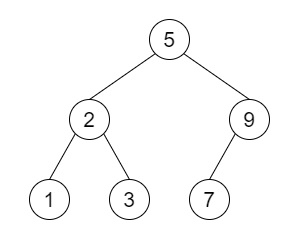# Serialize and Deserialize BST in Python

Suppose we want to design an algorithm to serialize and deserialize a binary search tree. Serialization is the process of converting something (data structure or object) into a sequence of bits so that it can be stored in a file or memory buffer, or transmitted across a network connection link. This can be reconstructed later that process is deserialization.

So, if the input is like [5,2,9,1,3,7], then the output will be Serialized output 5.2.9.1.3.7.N.N.N.N.N.N.N Deserialized output: 1, 2, 3, 5, 7, 9, (inorder traversal)To solve this, we will follow these steps −

• Define a function serialize() . This will take root

• res := a new list

• define one queue and insert root

• while queue is non-empty, do

• while queue is not empty, do

• current := queue

• insert current at the end of res

• delete first element from queue

• if current is non-zero, then

• come out from the loop

• if current is null, then

• come out from the loop

• if current.left is not null, then

• insert current.left at the end of queue

• otherwise,

• insert None at the end of queue

• if current.right is not null, then

• insert current.right at the end of queue

• otherwise,

• insert None at the end of queue

• s:= blank string

• for i in range 0 to size of res, do

• if res[i] is non-zero, then

• s := s concatenate res[i].data

• otherwise,

• s := s concatenate "N"

• if i is same as size of res -1, then

• come out from the loop

• s := s concatenate "."

• return s

• Define a function deserialize() . This will take data

• data := a list of parts after dividing the data using dot

• stack := a new list

• if data is same as 'N', then

• return None

• root := create a new node with data data

• insert root at the end of stack

• i := 1

• current := 0

• while i < size of data, do

• left:= False

• if data[i] is not same as 'N', then

• temp := create a new node with data data[i]

• stack[current].left := temp

• insert temp at the end of stack

• otherwise,

• stack[current].left := None

• i := i + 1

• if data[i] is not same as 'N', then

• temp := create a new node with data data[i]

• stack[current].right := temp

• insert temp at the end of stack

• otherwise,

• stack[current].right := None

• current := current + 1

• i := i + 1

• return root

## Example

Let us see the following implementation to get a better understanding −

Live Demo

class TreeNode:
def __init__(self, data, left = None, right = None):
self.data = data
self.left = left
self.right = right
def insert(temp,data):
que = []
que.append(temp)
while (len(que)):
temp = que
que.pop(0)
if (not temp.left):
if data is not None:
temp.left = TreeNode(data)
else:
temp.left = TreeNode(0)
break
else:
que.append(temp.left)
if (not temp.right):
if data is not None:
temp.right = TreeNode(data)
else:
temp.right = TreeNode(0)
break
else:
que.append(temp.right)
def make_tree(elements):
Tree = TreeNode(elements)
for element in elements[1:]:
insert(Tree, element)
return Tree
def print_tree(root):
#print using inorder traversal
if root is not None:
print_tree(root.left)
print(root.data, end = ', ')
print_tree(root.right)
class Codec:
def serialize(self, root):
res =[]
queue = [root]
while queue:
while True and queue:
current = queue
res.append(current)
queue.pop(0)
if current:
break
if not current:
break
if current.left:
queue.append(current.left)
else:
queue.append(None)
if current.right:
queue.append(current.right)
else:
queue.append(None)
s=""
for i in range(len(res)):
if res[i]:
s+=str(res[i].data)
else:
s+="N"
if i == len(res)-1:
break
s+="."
return s
def deserialize(self, data):
data = data.split(".")
stack = []
if data=='N':
return None
root = TreeNode(int(data))
stack.append(root)
i = 1
current = 0
while i <len(data):
left= False
if data[i] !='N':
temp = TreeNode(int(data[i]))
stack[current].left = temp
stack.append(temp)
else:
stack[current].left = None
i+=1
if data[i] !='N':
temp = TreeNode(int(data[i]))
stack[current].right = temp
stack.append(temp)
else:
stack[current].right = None
current+=1
i+=1
return root

ob = Codec()
root = make_tree([5,2,9,1,3,7])
ser = ob.serialize(root)
print('Serialization:',ser)
print_tree(ob.deserialize(ser))

## Input

[5,2,9,1,3,7]

## Output

Serialization: 5.2.9.1.3.7.N.N.N.N.N.N.N
1, 2, 3, 5, 7, 9,